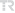## ON THE LINEAR CODES OVER THE RING Z(4)

COMMUNICATIONS FACULTY OF SCIENCES UNIVERSITY OF ANKARA-SERIES A1 MATHEMATICS AND STATISTICS, vol.68, no.1, pp.809-823, 2019 (ESCI)• Publication Type: Article / Article
• Volume: 68 Issue: 1
• Publication Date: 2019
• Doi Number: 10.31801/cfsuasmas.478655
• Journal Name:
• Journal Indexes: Emerging Sources Citation Index (ESCI), TR DİZİN (ULAKBİM)
• Page Numbers: pp.809-823
• Keywords: Cyclic codes, skew codes, DNA codes, MDS codes, CYCLIC CODES, CONSTRUCTION
• Ondokuz Mayıs University Affiliated: Yes

#### Abstract

Some results on linear codes over the ring Z(4) + uZ(4) + vZ(4); u(2) = u, v(2) = v; uv = vu = 0 in [6,7] are generalized to the ring D-t = Z(4) + v(1)Z(4) + ... + v(t)Z(4); v(i)(2) = v(i),v(i)v(j) = v(j)v(i) = 0 for i not equal j; 1 <= i, j <= t. A Gray map Phi(t) from D-t(n) to Z(4)((t+1)n) is defined. The Gray images of the cyclic, constacyclic and quasi-cyclic codes over Dt are determined. The cyclic DNA codes over D-t are introduced. The binary images of them are determined. The nontrivial automorphism on D-i for i = 2; 3; ..., t is given. The skew cyclic, skew constacyclic and skew quasi-cyclic codes over D-t are introduced. The Gray images of them are determined. The skew cyclic DNA codes over D-t are introduced. Moreover, some properties of MDS codes over D-t are discussed.